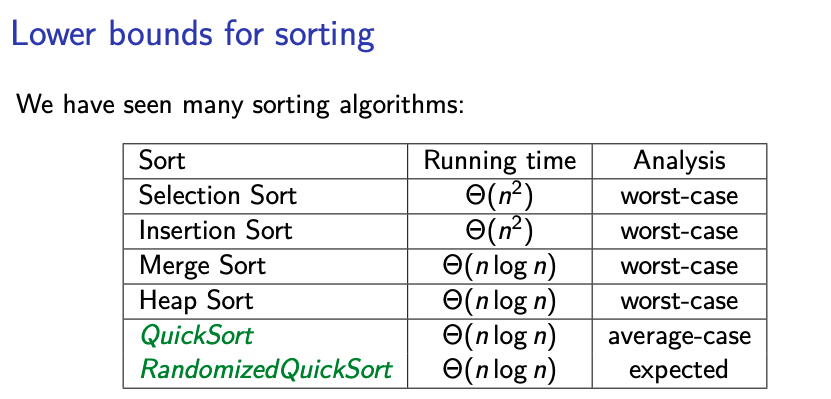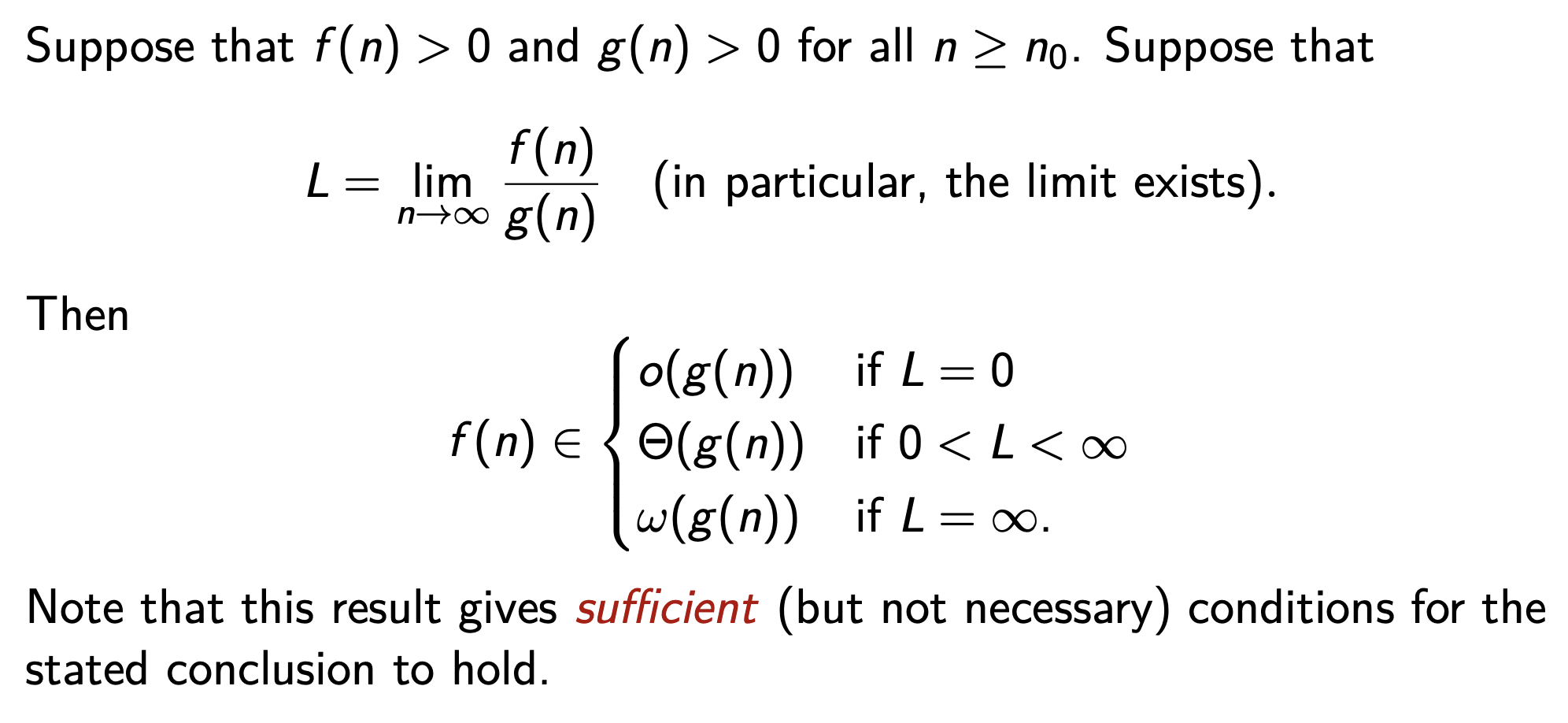# Asymptotic Analysis §

Learned in CS240, did a formal study of Big O.

### Smaller / Larger Asymptotic Bounds §

-notation: ( is asymptotically bounded above by if there exist constants and such that for all .

-notation: (f is asymptotically bounded below by g) if there exist constants and such that for all .

-notation: ( is asymptotically tightly bounded by ) if there exist constants and such that for all .

• This is usually what we talk about in Leetcode interviews

Do NOT get confused

For some reason, I used to think that was synonymous with “best case”, was the same as “worst case” , and was the “average case”. This is misleading. For each case, you can calculate .Maybe the confusion comes from the Sorting Algorithms page (yea…)

### Strictly Smaller / Larger Asymptotic Bounds §

-notation: (f is asymptotically strictly smaller than ) if for all constants , there exists a constant such that for all .

-notation: (f is asymptotically strictly larger than ) if for all constants , there exists a constant such that for all .

Notes

• Main difference to , is the quantifier for .
• Rarely proved from first principles.

### Algebra of Order Notations §

Identity rule:

Transitivity:

• If and then
• If and then

Maximum rules: Suppose that and for all . Then:

### Techniques for Order Notation §